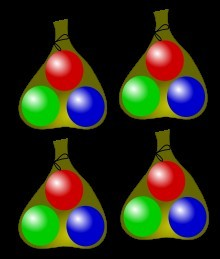Examples for business, study, careers, love, and more...
Examples :: Lesson Plans :: Example of Mathematics Lesson Plan:Multiplying Two Digit Numbers by One

# Example of Mathematics Lesson Plan:Multiplying Two Digit Numbers by OneFour bags of three marbles gives twelve marbles. There are also 3 sets consisting of 4 marbles of the same colour.
1. Objective/s:

Multiplies 2- digit numbers by 1- digit numbers with regrouping
2. Subject Matter:
1. Multiplying 2- digit numbers by 1- digit numbers with regrouping
2. Integration
Character Education: Observing measures to ensure clean, safe water for drinking
3. Science: Uses and sources of water for households

3. References/ Material/s:
1. Math handbook,
2. Charts

4. Procedure:
1. Drill
Flashcard drill on basic multiplication facts
2. Presentation/ Discussion:
3. Problem Statement

1. In a place Maunlad, there is a deep well for every 12 families. There are 8 deep wells in the whole barangay. How many families are there? Showing the process of dividing 2- digit numbers by 1- digit numbers by 1- digit numbers with regrouping

1. First, multiply the ones. 2x 8=16
2. Write 6 under the same column. Carry 1 over the tens column.
3. Then multiply the tens. 1x 8= 8. Add the carried number (1) to the product (8) hence, 1+8=9
4. Write 9 under the tens column. The complete answer is 96 families.
1. Practice exercises:
1. Find the product:
2.  ``` 25 × 3 ``` ``` 68 × 2 ``` ``` 49 × 4 ``` ``` 82 × 5 ``` ``` 17 × 4 ```
3. Do similar exercises in the textbook
2. Application:
1. Fixing skills
A family uses 55 buckets of water in one week. How many buckets of water are used in 4 weeks?
2. Science and Character Education Integration:
How do the households use water?
What are the sources of water? How can we make sure that the water we use is clean and safe?

3. Evaluation:

1. Find the product:
2.  ``` 34 × 3 ``` ``` 58 × 2 ``` ``` 26 × 4 ``` ``` 56 × 5 ``` ``` 48 × 3 ```